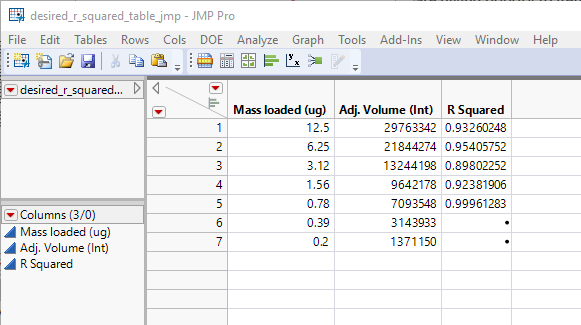Choose Language Hide Translation Bar
Highlighted

## How to update values in a for loop from bivariate plot

Hi all,

I'm a JMP newbie and can't figure this out for the life of me.

I have a simple data table with a response (Adjusted vol.) and one factor (Mass loaded). I want to find the r_squared used for lines that always start at the lowest mass loading and continue to make all possible lines (except for the one or two point cases). After each line drawn, I want the r_squared for each line to be put in my dataframe. But when I run my for loop, the r_squared for all rows fills with the that from the last made line. I think my semicolon after the bivariate plotting is stopping my for loop prematurely?

One overaraching question I have is how I can think about data stored in the output of a bivariate and that stored in the bivariate report.

I have attached my script and the desired data table output. My input datatable is just that without the r_squared column.

I would also like to get rid of the row# column but can't figure out how to make the lines without the local data filter.

Highlighted

## Re: How to update values in a for loop from bivariate plot

Here is a script that eliminates the need for Row # and the local filter.

``````dt = Current Data Table();

nrows = N Rows(dt);
dt << New Column("R Squared", Numeric, Continuous);

list_biv = list();

for (j = 1, j <= nrows-2,j++,
dt << clear rowstates;
dt << select where( Row() >= j);
dt << invert row selection;
dt << exclude;
biv = Bivariate(invisible,
Y( :Name( "Adj. Volume (Int)" ) ),
X( :Name( "Mass loaded (ug)" ) ),
Automatic Recalc( 1 ),
Fit Line( {Line Color( {212, 73, 88} )} )
);

RSquare = (Report(biv)["Linear Fit"][NumberColBox(1)]<<get) ;
:Name("R Squared")[j] = Rsquare;
);

dt << clear rowstates;``````

and I am not having any issue with the RSquare remaining the sameJim
Article Labels

There are no labels assigned to this post.